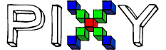# How can I get the CC signature instead of the decimal one

Hi, I’m trying to play around with the color code mode.I would like to get the highlighted number instead of the decimal which I get with the m_signature function.
Can you help me?
Butanium

Hi Butanium,

I’m not sure if this is possible - I don’t see anything in the docs about it. Maybe @edge has some info?

Cheers,
Jesse

Hello,
The highlighted number is in base-8 (octal) representation.

You can convert a decimal number to octal using the code in pixy2CCC.h:

The code that does this is below:

``````> // convert signature number to an octal string
>       for (i=12, j=0, flag=false; i>=0; i-=3)
>       {
>         d = (m_signature>>i)&0x07;
>         if (d>0 && !flag)
>           flag = true;
>         if (flag)
>           sig[j++] = d + '0';
>       }
>       sig[j] = '\0';
``````

Most users just figure out what number they are looking for (in decimal) and use that for comparison.

This site can convert from decimal to octal:

https://www.rapidtables.com/convert/number/decimal-to-octal.html

and vice-versa:

https://www.rapidtables.com/convert/number/octal-to-decimal.html

Hope this helps!

Edward

1 Like

Ok thx